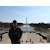# Introduction

A probability distribution is the mathematical function through which the probability of occurrence of different possible outcomes in an experiment can be calculated.

Some very common examples we can see for probability distributions are the rolling of a dice, marks of a particular subject of a class and occurrence of car accidents in a particular year. Type of distribution is useful when we need to know which outcomes are most likely, the spread of potential values, and the likelihood of different results.

Types of the probability distribution

While there are many different types of probability distributions, this blog will focus…

# Probability & Statistics for Beginners in Machine Learning : Part 2— Measures of Central Tendency

This series of posts will be for all those who face incredible challenges in grasping theories and concepts of Probability & Statistics when they begin their journey into Machine Learning. I have tried to cover most of the core concepts, and also illustrate their implementation in simple Python language in subsequent chapters.

# Introduction

A measure of central tendency is a summary statistic that represents the center point or typical value of a dataset. These measures indicate where most values in a distribution fall and are also referred to as the central location of a distribution. We can think of it as…

# Probability & Statistics for Beginners in Machine Learning : Part 1— Introduction

This series of posts will be for all those who face incredible challenges in grasping theories and concepts of Probability & Statistics when they begin their journey into Machine Learning. I have tried to cover most of the core concepts, and also illustrate their implementation in simple Python language in subsequent chapters.

# What is Statistics?

In very simple language, statistics is the science that deals with methodologies to gather, review, analyze and draw conclusions from data. With specific statistical tools in hand we can derive many key observations and make predictions from the data in hand.

# Types of Statistics

Broadly we can categorize statistics into two…